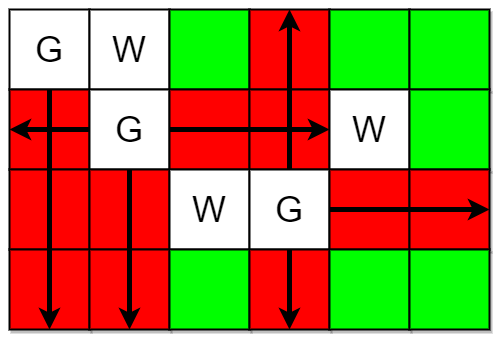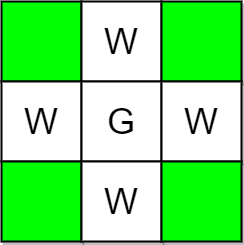##### Welcome to Subscribe On Youtube

Formatted question description: https://leetcode.ca/all/2257.html

# 2257. Count Unguarded Cells in the Grid

• Difficulty: Medium.
• Related Topics: Array, Matrix, Simulation.
• Similar Questions: Bomb Enemy, Available Captures for Rook.

## Problem

You are given two integers m and n representing a 0-indexed m x n grid. You are also given two 2D integer arrays guards and walls where guards[i] = [rowi, coli] and walls[j] = [rowj, colj] represent the positions of the ith guard and jth wall respectively.

A guard can see every cell in the four cardinal directions (north, east, south, or west) starting from their position unless obstructed by a wall or another guard. A cell is guarded if there is at least one guard that can see it.

Return** the number of unoccupied cells that are not guarded.**

Example 1:Input: m = 4, n = 6, guards = [[0,0],[1,1],[2,3]], walls = [[0,1],[2,2],[1,4]]
Output: 7
Explanation: The guarded and unguarded cells are shown in red and green respectively in the above diagram.
There are a total of 7 unguarded cells, so we return 7.


Example 2:Input: m = 3, n = 3, guards = [[1,1]], walls = [[0,1],[1,0],[2,1],[1,2]]
Output: 4
Explanation: The unguarded cells are shown in green in the above diagram.
There are a total of 4 unguarded cells, so we return 4.


Constraints:

• 1 <= m, n <= 105

• 2 <= m * n <= 105

• 1 <= guards.length, walls.length <= 5 * 104

• 2 <= guards.length + walls.length <= m * n

• guards[i].length == walls[j].length == 2

• 0 <= rowi, rowj < m

• 0 <= coli, colj < n

• All the positions in guards and walls are unique.

## Solution (Java, C++, Python)

• class Solution {
public int countUnguarded(int m, int n, int[][] guards, int[][] walls) {
char[][] matrix = new char[m][n];
int result = 0;
for (int i = 0; i <= guards.length - 1; i++) {
int guardRow = guards[i];
int guardCol = guards[i];
matrix[guardRow][guardCol] = 'G';
}
for (int i = 0; i <= walls.length - 1; i++) {
int wallRow = walls[i];
int wallCol = walls[i];
matrix[wallRow][wallCol] = 'W';
}
for (int i = 0; i <= guards.length - 1; i++) {
int currentRow = guards[i];
int currentCol = guards[i] - 1;
while (currentCol >= 0) {
if (matrix[currentRow][currentCol] != 'W'
&& matrix[currentRow][currentCol] != 'G') {
matrix[currentRow][currentCol] = 'R';
} else {
break;
}
currentCol--;
}
currentRow = guards[i];
currentCol = guards[i] + 1;
while (currentCol <= n - 1) {
if (matrix[currentRow][currentCol] != 'W'
&& matrix[currentRow][currentCol] != 'G') {
matrix[currentRow][currentCol] = 'R';
} else {
break;
}
currentCol++;
}
currentRow = guards[i] - 1;
currentCol = guards[i];
while (currentRow >= 0) {
if (matrix[currentRow][currentCol] != 'W'
&& matrix[currentRow][currentCol] != 'G') {
matrix[currentRow][currentCol] = 'R';
} else {
break;
}
currentRow--;
}
currentRow = guards[i] + 1;
currentCol = guards[i];
while (currentRow <= m - 1) {
if (matrix[currentRow][currentCol] != 'W'
&& matrix[currentRow][currentCol] != 'G') {
matrix[currentRow][currentCol] = 'R';
} else {
break;
}
currentRow++;
}
}
for (int i = 0; i <= m - 1; i++) {
for (int j = 0; j <= n - 1; j++) {
if (matrix[i][j] != 'R' && matrix[i][j] != 'G' && matrix[i][j] != 'W') {
result++;
}
}
}
return result;
}
}

############

class Solution {
public int countUnguarded(int m, int n, int[][] guards, int[][] walls) {
int[][] g = new int[m][n];
for (var e : guards) {
g[e][e] = 2;
}
for (var e : walls) {
g[e][e] = 2;
}
int[] dirs = {-1, 0, 1, 0, -1};
for (var e : guards) {
for (int k = 0; k < 4; ++k) {
int x = e, y = e;
int a = dirs[k], b = dirs[k + 1];
while (x + a >= 0 && x + a < m && y + b >= 0 && y + b < n && g[x + a][y + b] < 2) {
x += a;
y += b;
g[x][y] = 1;
}
}
}
int ans = 0;
for (var row : g) {
for (int v : row) {
if (v == 0) {
++ans;
}
}
}
return ans;
}
}

• class Solution:
def countUnguarded(
self, m: int, n: int, guards: List[List[int]], walls: List[List[int]]
) -> int:
g = [[None] * n for _ in range(m)]
for r, c in guards:
g[r][c] = 'g'
for r, c in walls:
g[r][c] = 'w'
for i, j in guards:
for a, b in [[0, -1], [0, 1], [1, 0], [-1, 0]]:
x, y = i, j
while (
0 <= x + a < m
and 0 <= y + b < n
and g[x + a][y + b] != 'w'
and g[x + a][y + b] != 'g'
):
x, y = x + a, y + b
g[x][y] = 'v'
return sum(not v for row in g for v in row)

############

# 2257. Count Unguarded Cells in the Grid
# https://leetcode.com/problems/count-unguarded-cells-in-the-grid

class Solution:
def countUnguarded(self, rows: int, cols: int, guards: List[List[int]], walls: List[List[int]]) -> int:
grid = [ * cols for _ in range(rows)]

for x, y in walls:
grid[x][y] = -1

def goLeft(x, y):
dy = y - 1
while dy >= 0 and grid[x][dy] != 2 and grid[x][dy] != -1:
grid[x][dy] = 2
dy -= 1

def goRight(x, y):
dy = y + 1
while dy < cols and grid[x][dy] != 3 and grid[x][dy] != -1:
grid[x][dy] = 3
dy += 1

def goTop(x, y):
dx = x - 1
while dx >= 0 and grid[dx][y] != 4 and grid[dx][y] != -1:
grid[dx][y] = 4
dx -= 1

def goBottom(x, y):
dx = x + 1
while dx < rows and grid[dx][y] != 5 and grid[dx][y] != -1:
grid[dx][y] = 5
dx += 1

guards.sort(key = lambda x : (-x, x))

for x, y in guards:
goLeft(x, y)

guards.sort(key = lambda x : (x, x))

for x, y in guards:
goRight(x, y)

guards.sort(key = lambda x : (-x, x))
for x, y in guards:
goTop(x, y)

guards.sort(key = lambda x : (x, x))
for x, y in guards:
goBottom(x, y)

for x, y in guards:
grid[x][y] = -1

res = 0

for x in range(rows):
for y in range(cols):
if grid[x][y] == 1:
res += 1

return res


• class Solution {
public:
int countUnguarded(int m, int n, vector<vector<int>>& guards, vector<vector<int>>& walls) {
int g[m][n];
memset(g, 0, sizeof(g));
for (auto& e : guards) {
g[e][e] = 2;
}
for (auto& e : walls) {
g[e][e] = 2;
}
int dirs = {-1, 0, 1, 0, -1};
for (auto& e : guards) {
for (int k = 0; k < 4; ++k) {
int x = e, y = e;
int a = dirs[k], b = dirs[k + 1];
while (x + a >= 0 && x + a < m && y + b >= 0 && y + b < n && g[x + a][y + b] < 2) {
x += a;
y += b;
g[x][y] = 1;
}
}
}
int ans = 0;
for (auto& row : g) {
ans += count(row, row + n, 0);
}
return ans;
}
};

• func countUnguarded(m int, n int, guards [][]int, walls [][]int) (ans int) {
g := make([][]int, m)
for i := range g {
g[i] = make([]int, n)
}
for _, e := range guards {
g[e][e] = 2
}
for _, e := range walls {
g[e][e] = 2
}
dirs := int{-1, 0, 1, 0, -1}
for _, e := range guards {
for k := 0; k < 4; k++ {
x, y := e, e
a, b := dirs[k], dirs[k+1]
for x+a >= 0 && x+a < m && y+b >= 0 && y+b < n && g[x+a][y+b] < 2 {
x, y = x+a, y+b
g[x][y] = 1
}
}
}
for _, row := range g {
for _, v := range row {
if v == 0 {
ans++
}
}
}
return
}

• function countUnguarded(m: number, n: number, guards: number[][], walls: number[][]): number {
const g: number[][] = Array.from({ length: m }, () => Array.from({ length: n }, () => 0));
for (const [i, j] of guards) {
g[i][j] = 2;
}
for (const [i, j] of walls) {
g[i][j] = 2;
}
const dirs: number[] = [-1, 0, 1, 0, -1];
for (const [i, j] of guards) {
for (let k = 0; k < 4; ++k) {
let [x, y] = [i, j];
let [a, b] = [dirs[k], dirs[k + 1]];
while (x + a >= 0 && x + a < m && y + b >= 0 && y + b < n && g[x + a][y + b] < 2) {
x += a;
y += b;
g[x][y] = 1;
}
}
}
let ans = 0;
for (const row of g) {
for (const v of row) {
ans += v === 0 ? 1 : 0;
}
}
return ans;
}



Explain:

nope.

Complexity:

• Time complexity : O(n).
• Space complexity : O(n).